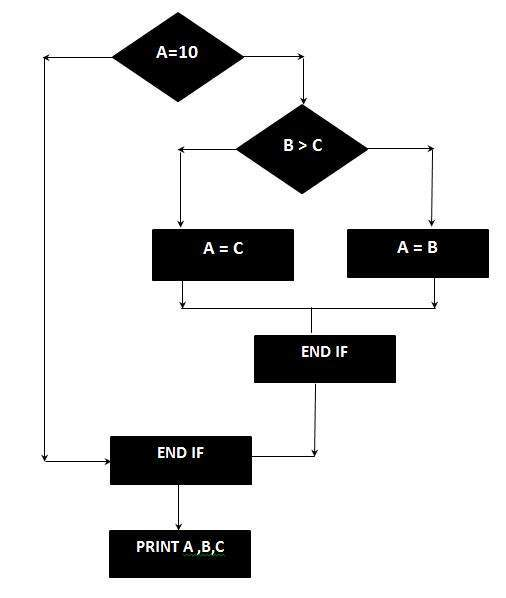# Cyclomatic Complexity

## What is Cyclomatic Complexity?

Cyclomatic complexity is a source code complexity measurement that is being correlated to a number of coding errors. It is calculated by developing a Control Flow Graph of the code that measures the number of linearly-independent paths through a program module.

Lower the Program's cyclomatic complexity, lower the risk to modify and easier to understand. It can be represented using the below formula:

```Cyclomatic complexity = E - N + 2*P
where,
E = number of edges in the flow graph.
N = number of nodes in the flow graph.
P = number of nodes that have exit points
```

## Example :

```IF A = 10 THEN
IF B > C THEN
A = B
ELSE
A = C
ENDIF
ENDIF
Print A
Print B
Print C
```

## FlowGraph:The Cyclomatic complexity is calculated using the above control flow diagram that shows seven nodes(shapes) and eight edges (lines), hence the cyclomatic complexity is 8 - 7 + 2 = 3